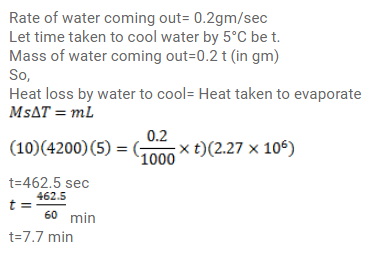# Indian style of cooling drinking water is to keep it in a pitcher having porous walls.Question:

Indian style of cooling drinking water is to keep it in a pitcher having porous walls. Water comes to the outer surface very slowly and evaporates. Most of the energy needed for evaporation is taken from the water itself and the water is cooled down. Assume that a pitcher is contains $10 \mathrm{~kg}$ of water and $0.2 \mathrm{~g}$ of water comes out per second. Assuming no backward heat transfer from the atmosphere to the water, calculate the time in which the temperature decreases by $5^{\circ} \mathrm{C}$. Specific heat capacity of water=4200 $\mathrm{J} / \mathrm{kg}^{\circ} \mathrm{C}$ and latent heat of vaporization of water $=2.27 \times 10^{6} \mathrm{~J} / \mathrm{kg}$.

Solution: